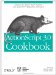# Recipe 6.2 Looping Through an Array

#### 6.2.1 Problem

You want to access or process all of the elements of an array in sequential order.

#### 6.2.2 Solution

Use a for loop that increments an index variable from 0 to Array.length - 1. Use the index to access each element in turn.

#### 6.2.3 Discussion

To access the values stored in the elements of an array, loop through the array's elements using a for loop. Because the first index of an array is 0, the index variable in the for statement should start at 0. The last index of an array is always 1 less than the length property of that array. Within the for statement, you can use the loop index variable within square brackets to access array elements. For example:

`myArray = ["a", "b", "c"]; for (var i = 0; i < myArray.length; i++) {   // Display the elements in the Output window.   trace("Element " + i + ": " + myArray[i]); }`

The looping index variable (i in the example code) should range from 0 to one less than the value of the length property. Remember that the last index of an array is always one less than its length.

Alternatively, you can also use a for statement that loops backward from Array.length - 1 to 0, decrementing by one each time. Looping backward is useful when you want to find the last matching element rather than the first (see Recipe 6.3). For example:

`myArray = ["a", "b", "c"]; for (var i = myArray.length - 1; i >= 0; i--){   // Display the elements in the Output window in reverse order.   trace("Element " + i + ": " + myArray[i]); }`

There are many examples in which you might want to loop through all the elements of an array. For example, by looping through an array containing references to movie clips, you can perform a particular action on each of the movie clips:

`for (var i = 0; i < myArray.length; i++){   // Move each movie clip one pixel to the right.   myArray[i]._x++; }`

You can improve the performance of a loop by storing the array's length in a variable rather than computing it during each loop iteration. For example:

`var len = myArray.length; for (var i = 0; i < len; i++){   // Move each movie clip one pixel to the right.   myArray[i]._x++; }`

This works as expected only if the length of the array does not change during the loop, which assumes that elements are not being added or removed.

See Recipe 5.5 for some correct and incorrect ways to loop through characters in a string. See Recipe 6.13 for details on enumerating elements of an associative array.ActionScript 3.0 Cookbook: Solutions for Flash Platform and Flex Application Developers
ISBN: 0596526954
EAN: 2147483647
Year: 2005
Pages: 425

Similar book on Amazon